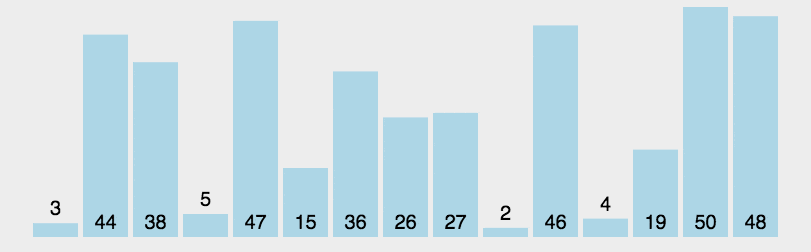• 累计撰写 869 篇文章
• 累计创建 55 个标签
• 累计收到 25 条评论

### 目 录CONTENT# 十大经典排序算法之六：快速排序2022-05-14 / 0 评论 / 0 点赞 / 139 阅读 / 1,945 字广告

# 六、快速排序

## 1. 算法步骤

1. 从数列中挑出一个元素，称为 “基准”（pivot）;
2. 重新排序数列，所有元素比基准值小的摆放在基准前面，所有元素比基准值大的摆在基准的后面（相同的数可以到任一边）。在这个分区退出之后，该基准就处于数列的中间位置。这个称为分区（partition）操作；
3. 递归地（recursive）把小于基准值元素的子数列和大于基准值元素的子数列排序；

1. 基本思想：（分治）
• 先从数列中取出一个数作为key值；
• 将比这个数小的数全部放在它的左边，大于或等于它的数全部放在它的右边；
• 对左右两个小数列重复第二步，直至各区间只有1个数。
1. 辅助理解：挖坑填数
• 初始时 i = 0; j = 9; key=72
• 由于已经将a中的数保存到key中，可以理解成在数组a上挖了个坑，可以将其它数据填充到这来。
• 从j开始向前找一个比key小的数。当j=8，符合条件，a = a ; i++ ; 将a挖出再填到上一个坑a中。
• 这样一个坑a就被搞定了，但又形成了一个新坑a，这怎么办了？简单，再找数字来填a这个坑。
• 这次从i开始向后找一个大于key的数，当i=3，符合条件，a = a ; j– ; 将a挖出再填到上一个坑中。
数组：72 - 6 - 57 - 88 - 60 - 42 - 83 - 73 - 48 - 85
0   1   2    3    4    5    6    7    8    9

• 此时 i = 3; j = 7; key=72
• 再重复上面的步骤，先从后向前找，再从前向后找。
• 从j开始向前找，当j=5，符合条件，将a挖出填到上一个坑中，a = a; i++;
• 从i开始向后找，当i=5时，由于i==j退出。
• 此时，i = j = 5，而a刚好又是上次挖的坑，因此将key填入a。
数组：48 - 6 - 57 - 88 - 60 - 42 - 83 - 73 - 88 - 85
0   1   2    3    4    5    6    7    8    9

• 可以看出a前面的数字都小于它，a后面的数字都大于它。因此再对a[0…4]和a[6…9]这二个子区间重复上述步骤就可以了。
数组：48 - 6 - 57 - 42 - 60 - 72 - 83 - 73 - 88 - 85
0   1   2    3    4    5    6    7    8    9


## 2. 动图演示## 3. JavaScript 代码实现

function quickSort(arr, left, right) {
var len = arr.length,
partitionIndex,
left = typeof left != 'number' ? 0 : left,
right = typeof right != 'number' ? len - 1 : right;

if (left < right) {
partitionIndex = partition(arr, left, right);
quickSort(arr, left, partitionIndex-1);
quickSort(arr, partitionIndex+1, right);
}
return arr;
}

function partition(arr, left ,right) {     // 分区操作
var pivot = left,                      // 设定基准值（pivot）
index = pivot + 1;
for (var i = index; i <= right; i++) {
if (arr[i] < arr[pivot]) {
swap(arr, i, index);
index++;
}
}
swap(arr, pivot, index - 1);
return index-1;
}

function swap(arr, i, j) {
var temp = arr[i];
arr[i] = arr[j];
arr[j] = temp;
}
functiion paritition2(arr, low, high) {
let pivot = arr[low];
while (low < high) {
while (low < high && arr[high] > pivot) {
--high;
}
arr[low] = arr[high];
while (low < high && arr[low] <= pivot) {
++low;
}
arr[high] = arr[low];
}
arr[low] = pivot;
return low;
}

function quickSort2(arr, low, high) {
if (low < high) {
let pivot = paritition2(arr, low, high);
quickSort2(arr, low, pivot - 1);
quickSort2(arr, pivot + 1, high);
}
return arr;
}


## 4. Python 代码实现

def quickSort(arr, left=None, right=None):
left = 0 if not isinstance(left,(int, float)) else left
right = len(arr)-1 if not isinstance(right,(int, float)) else right
if left < right:
partitionIndex = partition(arr, left, right)
quickSort(arr, left, partitionIndex-1)
quickSort(arr, partitionIndex+1, right)
return arr

def partition(arr, left, right):
pivot = left
index = pivot+1
i = index
while  i <= right:
if arr[i] < arr[pivot]:
swap(arr, i, index)
index+=1
i+=1
swap(arr,pivot,index-1)
return index-1

def swap(arr, i, j):
arr[i], arr[j] = arr[j], arr[i]


## 5. Go 代码实现

func quickSort(arr []int) []int {
return _quickSort(arr, 0, len(arr)-1)
}

func _quickSort(arr []int, left, right int) []int {
if left < right {
partitionIndex := partition(arr, left, right)
_quickSort(arr, left, partitionIndex-1)
_quickSort(arr, partitionIndex+1, right)
}
return arr
}

func partition(arr []int, left, right int) int {
pivot := left
index := pivot + 1

for i := index; i <= right; i++ {
if arr[i] < arr[pivot] {
swap(arr, i, index)
index += 1
}
}
swap(arr, pivot, index-1)
return index - 1
}

func swap(arr []int, i, j int) {
arr[i], arr[j] = arr[j], arr[i]
}


## 6. C++版

 //严蔚敏《数据结构》标准分割函数
Paritition1(int A[], int low, int high) {
int pivot = A[low];
while (low < high) {
while (low < high && A[high] >= pivot) {
--high;
}
A[low] = A[high];
while (low < high && A[low] <= pivot) {
++low;
}
A[high] = A[low];
}
A[low] = pivot;
return low;
}

void QuickSort(int A[], int low, int high) //快排母函数
{
if (low < high) {
int pivot = Paritition1(A, low, high);
QuickSort(A, low, pivot - 1);
QuickSort(A, pivot + 1, high);
}
}


## 7. java版

public static void quickSort(int a[],int l,int r){
if(l>=r)
return;

int i = l; int j = r; int key = a[l];//选择第一个数为key

while(i<j){

while(i<j && a[j]>=key)//从右向左找第一个小于key的值
j--;
if(i<j){
a[i] = a[j];
i++;
}

while(i<j && a[i]<key)//从左向右找第一个大于key的值
i++;

if(i<j){
a[j] = a[i];
j--;
}
}
//i == j
a[i] = key;
quickSort(a, l, i-1);//递归调用
quickSort(a, i+1, r);//递归调用
}

• key值的选取可以有多种形式，例如中间数或者随机数，分别会对算法的复杂度产生不同的影响。
0
1.2.广告
• 0
1.2.